﻿ NLVM 9 - 12 - Number & Operations Manipulatives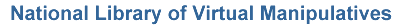Number & Operations (Grades 9 - 12)

Virtual manipulatives for Number & Operations, grades 9 - 12.Abacus – An electronic abacus that can be used to do arithmetic.Circle 0 – A puzzle involving adding positive and negative integers to sum to zero.Circle 21 – A puzzle involving adding positive and negative integers to sum to twenty one.Circle 3 – A puzzle involving adding positive real numbers to sum to three.Circle 99 – A puzzle involving adding positive and negative integers to sum to ninety nine.Conway's Game of Life – Discover the rules that determine change in these simulations.Counting All Pairs – Create a path that sets up a one-to-one correspondence between the counting numbers and infinite sets of ordered pairs of integers.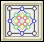Diffy – Solve an interesting puzzle involving the differences of given numbers.Dueling Calculators – Visualize a dramatic simulation of the effect of propagating rounding errors.Fibonacci Sequence – Explore the Fibonacci sequence and the golden ratio.Fractions - Adding – Illustrates what it means to find a common denominator and combine.Fractions - Equivalent – Illustrates relationships between equivalent fractions.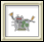Function Machine – Explore the concept of functions by putting values into this machine and observing its output.Golden Rectangle – Illustrates iterations of the Golden Section.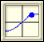Grapher – A tool for graphing and exploring functions.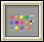Mastermind – Use inference and logic to play a game and guess a hidden pattern of pegs.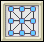Number Puzzles – Solve puzzles involving arranging numbers on a diagram so that they add up to a given value.Pascal's Triangle – Explore patterns created by selecting elements of Pascal's triangle.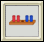Peg Puzzle – Win this game by moving the pegs on the left past the pegs on the right.Percentages – Discover relationships between fractions, percents, and decimals.Rational Numbers Triangle – Explore a triangular array that contains every positive rational number exactly once.Sieve of Eratosthenes – Relate number patterns with visual patterns.Tight Weave – Visualize the creation of the Sierpinski Carpet, an iterative geometric pattern that resembles a woven mat.Turtle Geometry – Explore numbers, shapes, and logic by programming a turtle to move.Venn Diagrams – Investigate common features of sets.